Including my content from SQLBlog.com and some from SQLPerformance.com

## Sunday 27 February 2011

### SQL Server Bug: Slow T-SQL Sums and Averages

SQL Server Bug: Slow T-SQL Sums and Averages

It’s a curious thing about SQL that the `SUM` or `AVG` of no items (an empty set) is not zero, it’s `NULL`.

In this post, you’ll see how this means your `SUM` and `AVG` calculations might run at half speed, or worse. As usual though, this entry is not so much about the result, but the journey we take to get there.

## Average of no rows

Take a look at this query written against the AdventureWorks sample database:Notice the explicit `NULL`. The optimizer can see that this query contains a contradiction, `1=0`, so there’s no need to access the table at all. The query will always compute an average over an empty set of rows, and the answer will always be `NULL`.

The Constant Scan operator constructs a row in memory without accessing any base tables, or needing to acquire any locks.

## Normal sum and average calculations

Let’s look at a less trivial example: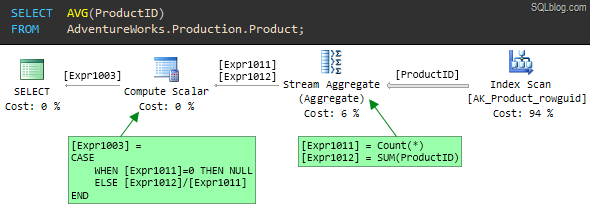Following the flow of data from right to left, the first thing to notice is that the optimizer has decided that the cheapest way to find all the `ProductID` values from the table is to scan the non-clustered index `AK_Product_rowguid`.

As the index name suggests, this is a nonclustered index on the `row_guid` column. Because `ProductID` is the unique clustered index for this table, the nonclustered index also includes the `ProductID` values at the leaf level of the index.

The nonclustered index is much narrower than the clustered index, because the latter contains all in-row table columns at the leaf level. In other words, all `ProductID` values in the table are stored much more densely in the nonclustered index. The higher density (and smaller size) of the nonclustered index means it is the lowest cost access method if all we need are product ids.

### Calculation details

The output from the Index Scan operator is a stream of rows containing a single column – `ProductID`. The Stream Aggregate consumes all the rows from the Index Scan to compute two values:

1. The number of rows encountered (`Count(*)`)
2. The `SUM` of the values (`SUM(ProductID)`).

The output from the Stream Aggregate is a single row with two columns:

1. The result of the count is labelled `[Expr1011]`
2. The result of the sum is labelled `[Expr1012]`

The final step to compute the average of `ProductID` values is performed by the Compute Scalar. It computes a single expression (`[Expr1003]`) using a `CASE` statement, which first checks to see if the count of rows (`[Expr1011]`) is zero.

If the count is zero, the result is defined to be `NULL`, otherwise the average is computed as the `SUM` divided by the `COUNT`: `[Expr1012] / [Expr1011]`.

Note that the left-to-right evaluation guarantee of the `CASE` statement ensures that a divide-by-zero error never occurs.

The plan for the same query using `SUM` instead of `AVG` is very similar:The only difference is in the Compute Scalar. The `ELSE` clause now returns the `SUM` if any rows were counted, rather than the average.

### Counting rows

The interesting thing about both `SUM` and `AVG` examples is that the optimizer produces a plan which counts the rows as well as summing them. In both cases, the count is needed so the Compute Scalar can produce a `NULL` if no rows are aggregated.

One might think that it would be more efficient for the Stream Aggregate to just set a bit flag indicating whether zero or more rows were seen, rather than counting all of them, but that is not the way it works.

The question is, does this extra counting operation add any significant overhead to the query execution? The answer might surprise you — and it isn’t as simple as it seems.

## Demo

To demonstrate the effect, we will need a larger table than the AdventureWorks database can provide.

``````USE master;
GO
IF DB_ID(N'SumTest') IS NOT NULL
BEGIN
ALTER DATABASE SumTest
SET SINGLE_USER
WITH ROLLBACK IMMEDIATE;

DROP DATABASE SumTest;
END;
GO
CREATE DATABASE SumTest
COLLATE LATIN1_GENERAL_CS_AS;
GO
ALTER DATABASE SumTest
MODIFY FILE
(
NAME = N'SumTest',
SIZE = 1GB,
MAXSIZE = 1GB
);
GO
ALTER DATABASE SumTest
MODIFY FILE
(
NAME = N'SumTest_log',
SIZE = 256MB,
MAXSIZE = 256MB
);
GO
USE SumTest;
GO
ALTER DATABASE SumTest SET
ALLOW_SNAPSHOT_ISOLATION OFF;

ALTER DATABASE SumTest SET
AUTO_CLOSE OFF,
AUTO_SHRINK OFF,
AUTO_CREATE_STATISTICS ON,
AUTO_UPDATE_STATISTICS ON,
AUTO_UPDATE_STATISTICS_ASYNC OFF,
PARAMETERIZATION SIMPLE,
RECOVERY SIMPLE,
RESTRICTED_USER;
GO
CREATE TABLE dbo.Test
(
id bigint IDENTITY (1,1) NOT NULL,

CONSTRAINT [PK dbo.Test (id)]
PRIMARY KEY CLUSTERED (id),
);
GO
-- Minimally-logged in 2008 onward
INSERT dbo.Test
WITH (TABLOCKX)
SELECT TOP (100000)
padding = REPLICATE(CHAR(65 + ([Data].n % 26)), 7999)
FROM
(
SELECT TOP (100000)
n = ROW_NUMBER() OVER (
ORDER BY (SELECT 0))
FROM master.sys.columns AS C1
CROSS JOIN master.sys.columns AS C2
CROSS JOIN master.sys.columns AS C3
ORDER BY
n ASC
) AS [Data]
ORDER BY
Data.n ASC;
``````

That script creates a new database containing a single table with 100,000 very wide rows.

The `id` column is `bigint IDENTITY`, and the `padding` column is defined as `char(7999)`. The data looks like this: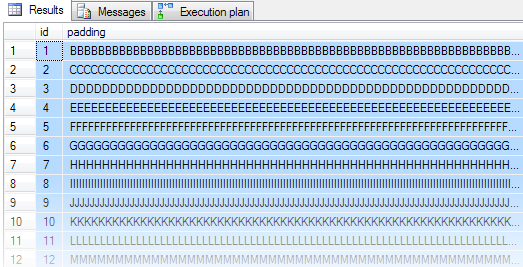To make the test CPU-intensive, we’ll calculate `CHECKSUM` values for the `REVERSE` of the strings in the padding column, and `SUM` the result.

A natural way to write that query follows. The convert to `bigint` is to avoid an arithmetic overflow.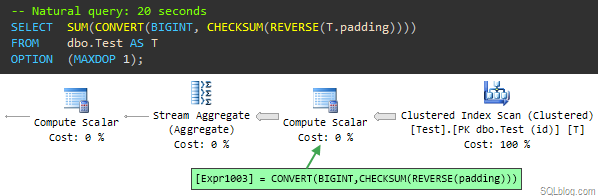The plan is essentially the same as the ones seen previously, with the addition of an extra Compute Scalar to calculate the new processing-intensive expression.

This query runs for around 20 seconds, even with the table data entirely in memory.

If we run a similar query that uses a `MIN` or `MAX` aggregate instead of `SUM`, the query returns results in 10 seconds — half the time:This is a very similar plan, but without the final Compute Scalar that decides whether to return `NULL` or not as seen in the `SUM` and `AVG` plans.

## Explanation

Some people might compare the `MAX` and `SUM` queries and conclude that the difference in execution time is down to the missing Compute Scalar.

That would be wrong because the 10 seconds of extra CPU time cannot possibly be caused by a simple scalar computation operating on the single row present at that stage of the plan.

In fact, the problem is in the Stream Aggregate, which in the `SUM` plan is computing two expressions:

``````[Expr1009] = COUNT_BIG([Expr1003])
[Expr1010] = SUM([Expr1003])
``````

`[Expr1003]` is our compute-intensive calculation, contained in the preceding Compute Scalar.

### Compute Scalars

You would think that by the time the Stream Aggregate processes a row, the preceding Compute Scalar has already assigned a result value to `[Expr1003]` for that row, right?

Wrong! Compute Scalars are implemented a little differently from other plan operators. In general, a Compute Scalar operator does not perform the calculation as rows flow through it; the work is deferred until a later plan operator needs the computed value.

In most cases, this is a useful optimization because rows may be eliminated by a later join or filter before the result value is really needed.

In this case, the Stream Aggregate is the first downstream operator to need the value of `[Expr1003]`, and it references it twice: Once for the count, and once for the sum.

The shocking truth is that the plan evaluates the whole `CHECKSUM(REVERSE(padding))` expression twice. One of those times it is just counting rows so it knows whether to return `NULL` later on or not.

### The bug exposed, and a ‘workaround’

We can verify this is the case by writing the query a bit differently, to ensure that the expensive expression is calculated once before the Stream Aggregate receives the row:Now, the expensive expression is calculated in a Constant Scan operator rather than a Compute Scalar.

The Stream Aggregate still references the expression twice, once for the `COUNT` and once for the `SUM`, but it is counting and summing the result of the calculation, not causing the calculation to be performed twice.

This query form produces the same result as the 20-second query, but does so in only 10 seconds. Both tests push a single CPU to 100% utilization, in case you were wondering about that.

To really make the point, consider this query:

``````SELECT
FROM dbo.Test AS T
OPTION
(MAXDOP 1);
``````

In the resulting plan, the Stream Aggregate references the `CHECKSUM(REVERSE(padding))` expression four times, and the query runs for 40 seconds at 100% CPU!

You might also wonder why the rewrite uses an `OUTER APPLY` instead of `CROSS APPLY`. The reason is that with a `CROSS APPLY`, the optimizer sees through the trick and transforms the query plan to exactly the same form as before, and the query runs for 20 seconds as a result.

The `OUTER APPLY` is enough the defeat an optimizer transformation rule, resulting in a plan featuring a left outer join. Before you go off to rewrite all your `SUMs` and `AVGs` using `OUTER APPLY`, consider:

1. The `OUTER APPLY` trick is a hack that depends on implementation details. It might not work tomorrow.
2. This problem only occurs if the first operator to need an expression result references it more than once.
3. This bug is only noticeable if the expression is expensive.

This is a confirmed bug in SQL Server. The good news is that it has been fixed for SQL Server 2012. The bug was originally reported on Connect but that information was lost when Microsoft suddenly retired that site.

### Indexed computed column

Finally for today, I want to show you another good reason not to use the `OUTER APPLY` pattern as a fix.

Let’s assume we don’t know about this problem, and need to optimize the original `CHECKSM(REVERSE(padding))` query.

The obvious solution is to add a computed column, and index it:

``````-- Add computed column
ALTER TABLE dbo.Test

-- Index it
CREATE INDEX
[IX dbo.Test Expr1003]
ON dbo.Test
([Expr1003])
WITH
(
FILLFACTOR = 100,
MAXDOP = 1,
SORT_IN_TEMPDB = ON
);
``````

Note the new computed column is not persisted, so it doesn’t require any extra storage in the table itself, and does not fragment the clustered index.

Re-running the natural form of the query: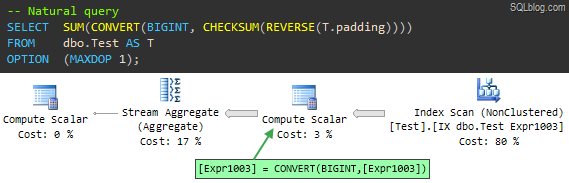It now completes in 30ms — quite an improvement over 20,000ms.

The Stream Aggregate still performs the two `SUM` and `COUNT` operations, but now the expression result is available directly from the index. It isn’t calculated at all, let alone twice.

If we re-run the `OUTER APPLY` query, we find that it does not use the index and uses exactly the same plan as it did previously, again taking 10 seconds to run.

Ironically, it fails to take advantage of the index for the same reason it previously performed better: The rewrite trick prevents the optimizer from matching the computed expression to the index.

Attempting to force the use of the index results in a very unhappy plan: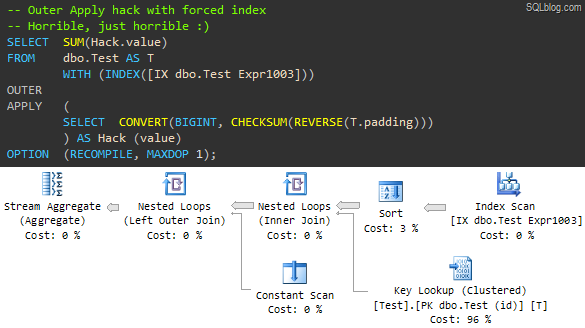The optimizer still can’t make the transformation to use the pre-computed value from our index. It honours the hint in the only way it can, by using the index to retrieve `id` column values (the unique clustering key included in the non-clustered index).

It then performs a Key Lookup for every row into the clustered index to retrieve the `padding` column, and finally performs the expensive calculation once per row in the Constant Scan.

The Sort operator is there to ‘optimize’ the lookups. By pre-sorting on the clustering key (`id`) it hopes to turn the normally random I/O associated with a lookup into sequential I/O.

With the data all in memory, this plan still executes in ten seconds, but if the data needed to be fetched in from disk…well you try it.

So, how important is this bug? Well, it depends. You would have to take quite a deep look into each one of your production query plans to see how often a plan operator references a Compute Scalar expression more than once, where the expression is expensive to compute.

That’s probably not practical (though you could write some XQuery to search the plan cache for examples I suppose) but it is something to be aware of, just in case you come across a query that performs much slower than you might expect.

Anyway, the point of this post is once again that deep internals knowledge can sometimes come in very useful.